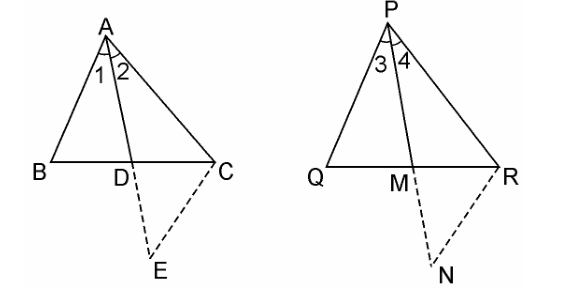Guru

# Sides AB and AC and median AD of a triangle ABC are respectively proportional to sides PQ and PR and median PM of another triangle PQR. Show that ΔABC ~ ΔPQR. Q.14

• 0

What is the best method to solve the problem of Exercise 6.3 of triangles, how to solve this problem in easy way Sides AB and AC and median AD of a triangle ABC are respectively proportional to sides PQ and PR and median PM of another triangle PQR. Show that ΔABC ~ ΔPQR.

Share

1. Given: Two triangles ΔABC and ΔPQR in which AD and PM are medians such that;

We have to prove, ΔABC ~ ΔPQR

Let us construct first: Produce AD to E so that AD = DE. Join CE, Similarly produce PM to N such that PM = MN, also Join RN.In ΔABD and ΔCDE, we have

BD = DC [Since, AP is the median]

and, ∠ADB = ∠CDE [Vertically opposite angles]

∴ ΔABD ≅ ΔCDE [SAS criterion of congruence]

⇒ AB = CE [By CPCT] …………………………..(i)

Also, in ΔPQM and ΔMNR,

PM = MN [By Construction.]

QM = MR [Since, PM is the median]

and, ∠PMQ = ∠NMR [Vertically opposite angles]

∴ ΔPQM = ΔMNR [SAS criterion of congruence]

⇒ PQ = RN [CPCT] ………………………………(ii)

Now, AB/PQ = AC/PR = AD/PM

From equation (i) and (ii),

⇒ CE/RN = AC/PR = 2AD/2PM

⇒ CE/RN = AC/PR = AE/PN [Since 2AD = AE and 2PM = PN]

∴ ΔACE ~ ΔPRN [SSS similarity criterion]

Therefore, ∠2 = ∠4

Similarly, ∠1 = ∠3

∴ ∠1 + ∠2 = ∠3 + ∠4

⇒ ∠A = ∠P …………………………………………….(iii)

Now, in ΔABC and ΔPQR, we have